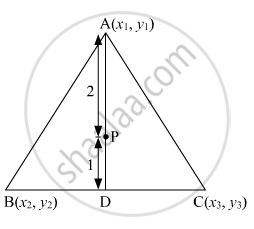Advertisement Remove all ads

# The points A ( x 1 , y 1 ) , B ( x 2 , y 2 ) , C ( x 3 , y 3 ) are the vertices of ABC . (i) The median from A meets BC at D . Find the coordinates of the point D. - Mathematics

Answer in Brief

The points  $A \left( x_1 , y_1 \right) , B\left( x_2 , y_2 \right) , C\left( x_3 , y_3 \right)$   are the vertices of  ΔABC .
(i) The median from meets BC at D . Find the coordinates of the point  D.
(ii) Find the coordinates of the point on AD such that AP : PD  = 2 : 1.
(iii) Find the points of coordinates Q and on medians BE and CF respectively such thatBQ : QE = 2 : 1 and CR : RF = 2 : 1.
(iv) What are the coordinates of the centropid of the triangle ABC

Advertisement Remove all ads

#### Solution

(i) Median AD of the triangle will divide the side BC in two equal parts.Therefore, D is the midpoint of side BC.
Coordinates of D are $\left( \frac{x_2 + x_3}{2}, \frac{y_2 + y_3}{2} \right)$

(ii)THe point P divided the side AD in the ratio 2: 1.
Coordinates of P are $\left( \frac{2 \times \left( \frac{x_2 + x_3}{2} \right) + 1 \times x_1}{2 + 1}, \frac{2 \times \left( \frac{y_2 + y_3}{2} \right) + 1 \times y_1}{2 + 1} \right) = \left( \frac{x_1 + x_2 + x_3}{3}, \frac{y_1 + y_2 + y_3}{3} \right)$

(iii)
Median BE of the triangle will divide the side AC in two equal parts.
Therefore, E is the midpoint of side AC.
Coordinates of E are $\left( \frac{x_1 + x_3}{2}, \frac{y_1 + y_3}{2} \right)$ The point Q divided the side BE in the ratio 2: 1.
Coordinates of Q are $\left( \frac{2 \times \left( \frac{x_1 + x_3}{2} \right) + 1 \times x_2}{2 + 1}, \frac{2 \times \left( \frac{y_1 + y_3}{2} \right) + 1 \times y_2}{2 + 1} \right) = \left( \frac{x_1 + x_2 + x_3}{3}, \frac{y_1 + y_2 + y_3}{3} \right)$

Similarly, Coordinates of Q are R are $\left( \frac{x_1 + x_2 + x_3}{3}, \frac{y_1 + y_2 + y_3}{3} \right)$

(iv)
The points P, Q and R coincides and is the centroid of the triangle ABC.
So, coordinates of the centroid is $\left( \frac{x_1 + x_2 + x_3}{3}, \frac{y_1 + y_2 + y_3}{3} \right)$

Is there an error in this question or solution?
Advertisement Remove all ads

#### APPEARS IN

RD Sharma Class 10 Maths
Chapter 6 Co-Ordinate Geometry
Exercise 6.3 | Q 60 | Page 31
Advertisement Remove all ads

#### Video TutorialsVIEW ALL 

Advertisement Remove all ads
Share
Notifications

View all notifications

Forgot password?